## RS Aggarwal Class 7 Solutions Chapter 15 Properties of Triangles Ex 15B

These Solutions are part of RS Aggarwal Solutions Class 7. Here we have given RS Aggarwal Solutions Class 7 Chapter 15 Properties of Triangles Ex 15B.

Other Exercises

Question 1.
Solution:
In ∆ABC,
∠A = 75°, ∠B = 45°
side BC is produced to D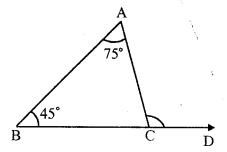forming exterior ∠ ACD
Exterior ∠ACD = ∠A + ∠B (Exterior angle is equal to sum of its interior opposite angles)
= 75° + 45° = 120°

Question 2.
Solution:
In ∆ABC, BC is produced to D forming an exterior angle ACD
∠ B = 68°, ∠ A = x°, ∠ ACB = y° and ∠ACD = 130°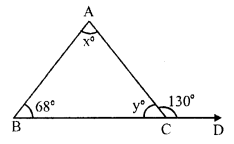In triangle,
Exterior angles is equal to sum of its interior opposite angles
∠ACD = ∠A + ∠B
⇒ 130° = x + 68°
⇒ x = 130° – 68° = 62°
But ∠ACB + ∠ACD = 180° (Linear pair)
⇒ y + 130° = 180°
⇒ y = 180° – 130° = 50°
Hence x = 62° and y = 50°

Question 3.
Solution:
In ∆ABC, side BC is produced to D forming exterior angle ACD.
∠ACD = 65°, ∠A = 32°
∠B = x, ∠ACB = y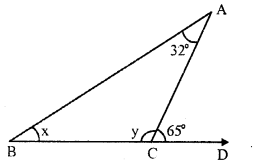In a triangle, the exterior angles is equal to the sum of its interior opposite angles
∠ACD = ∠A + ∠B
⇒ 65° = 32° + x
⇒ x = 65° – 32° = 33°
But ∠ ACD + ∠ ACB = 180° (Linear pair)
⇒ 65° + y = 180°
⇒ y = 180°- 65° = 115°
x = 33° and y = 115°

Question 4.
Solution:
In ∆ABC, side BC is produced to D forming exterior ∠ ACD∠ACD = 110°, and ∠A : ∠B = 2 : 3
In a triangle, exterior angles is equal to the sum of its interior opposite angles
⇒ ∠ACD = ∠A + ∠B
⇒ ∠A + ∠B = 110°
But ∠A : ∠B = 2 : 3But ∠A + ∠B + ∠C = 180° (sum of angles of a triangle)
⇒ 44° + 66° + ∠C = 180°
⇒ 110° + ∠C = 180°
⇒ ∠C = 180° – 110° = 70°
Hence ∠ A = 44°, ∠ B = 66° and ∠ C = 70°

Question 5.
Solution:
In ∆ABC, side BC is produced to forming exterior angle ACD.
∠ACD = 100° and ∠A = ∠B
Exterior angle of a triangle is equal to the sum of its interior opposite angles.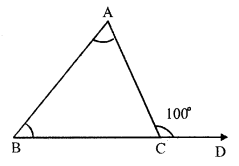∠ACD = ∠A + ∠B But ∠A = ∠B
∠A + ∠A = ∠ACD = 100°
⇒ 2 ∠A = 100°
⇒ ∠A = 50°
∠B = ∠A = 50°
But ∠A + ∠B + ∠ ACB = 180° (sum of angles of a triangle)
⇒ 50° + 50° + ∠ ACB = 180°
⇒ 100° + ∠ ACB = 180°
⇒ ∠ ACB = 180° – 100° = 80°
Hence ∠ A = 50°, ∠ B = 50° and ∠ C = 80°

Question 6.
Solution:
In ∆ABC, side BC is produced to D From D, draw a line meeting AC at E so that ∠D = 40°
∠A = 25°, ∠B = 45°
In ∆ABC,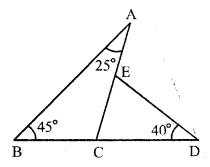Exterior ∠ACD = ∠A + ∠B = 25° + 45° = 70°
Again, in ∆CDE,
Exterior ∠ AED = ∠ ECD + ∠ D = ∠ACD + ∠D = 70° + 40° = 110°
Hence ∠ACD = 70° and ∠AED = 110°

Question 7.
Solution:
In ∆ABC, sides BC is produced to D and BA to E
∠CAD = 50°, ∠B = 40° and ∠ACB = 100°
∠ ACB + ∠ ACD = 180° (Linear pair)
⇒ 100° + ∠ ACD = 180°
⇒ ∠ ACD = 180° – 100° = 80°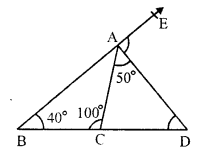In ∆ACD,
∠ CAD + ∠ ACD + ∠ ADC = 180° (sum of angles of a triangle)
⇒ 50° + 80° + ∠ ADC = 180°
⇒ 130° + ∠ ADC = 180°
⇒ ∠ ADC = 180° – 130° = 50°
Now, in ∆ABD, BA is produced to E
Exterior ∠DAE = ∠ACD + ∠ADC = 80° + 50° = 130°
Hence ∠ ACD = 80°, ∠ ADC = 50° and ∠DAE = 130°

Question 8.
Solution:
In ∆ABC, BC is produced to D forming exterior ∠ ACD∠ACD = 130°, ∠A = y°, ∠B = x° and ∠ACB = z°.
x : y = 2 : 3
Now, in ∆ABC,
Exterior ∠ACD = ∠A + ∠B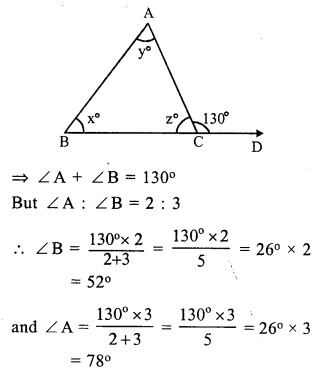But ∠A + ∠B + ∠ACB = 180° (sum of angles of a triangle)
⇒ 78° + 52° + ∠ACB = 180°
⇒ 130° + ∠ACB = 180°
⇒ ∠ACB = 180° – 130°
⇒ ∠ACB = 50°
⇒ ∠ = 50°
Hence x = 52°, y = 78° and z = 50°

Hope given RS Aggarwal Solutions Class 7 Chapter 15 Properties of Triangles Ex 15B are helpful to complete your math homework.

If you have any doubts, please comment below. Learn Insta try to provide online math tutoring for you.